# Step 1 Step 2 Step 3 plus add

• Slides: 41Step 1 Step 2 Step 3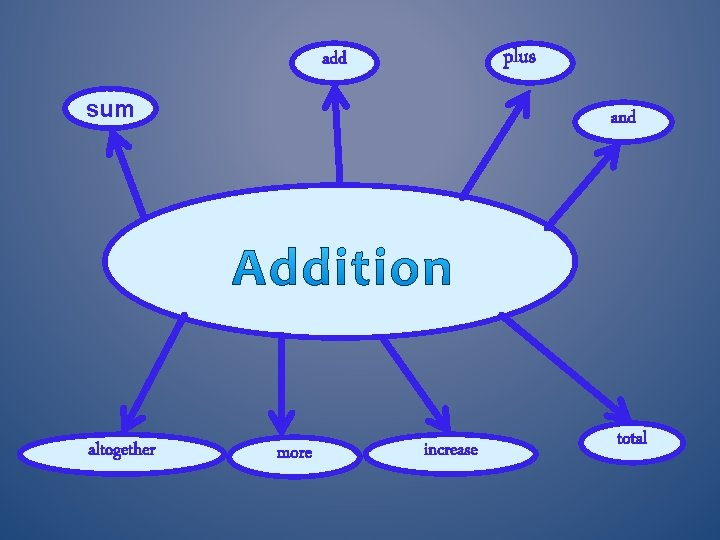plus add sum altogether and more increase total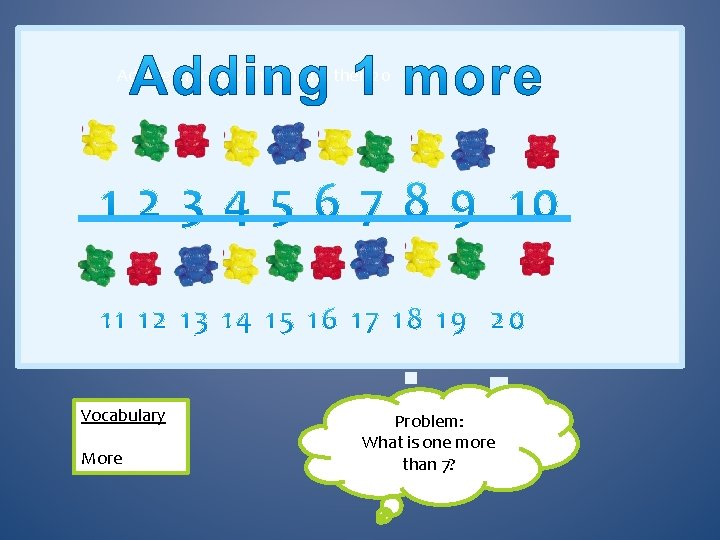Adding 1 more within 10 and then 20 Vocabulary More Problem: What is one more than 7?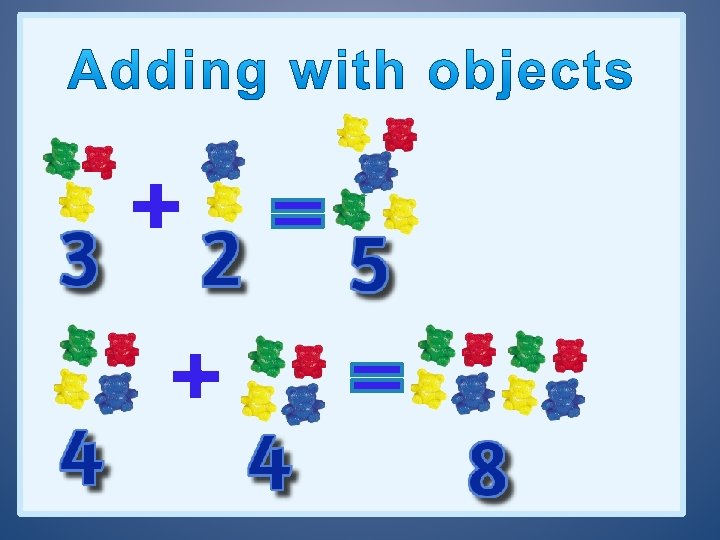Start at 3 and make 4 jumps. 0 1 2 3 4 5 7 6 Start at 2 and make 8 jumps. 0 1 2 3 4 5 6 7 8 9 10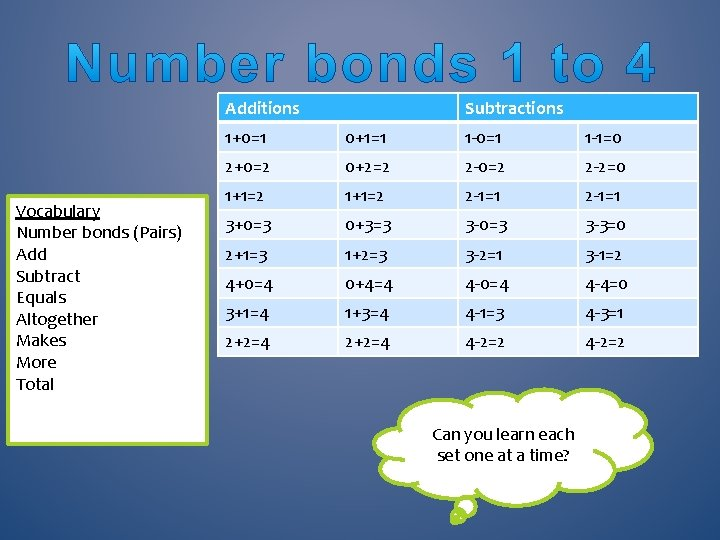Additions Vocabulary Number bonds (Pairs) Add Subtract Equals Altogether Makes More Total Subtractions 1+0=1 0+1=1 1 -0=1 1 -1=0 2+0=2 0+2=2 2 -0=2 2 -2=0 1+1=2 2 -1=1 3+0=3 0+3=3 3 -0=3 3 -3=0 2+1=3 1+2=3 3 -2=1 3 -1=2 4+0=4 0+4=4 4 -0=4 4 -4=0 3+1=4 1+3=4 4 -1=3 4 -3=1 2+2=4 4 -2=2 Can you learn each set one at a time?Additions Subtractions 5+0=5 0+5=5 5 -0=5 5 -5=0 4+1=5 1+4=5 5 -1=4 5 -4=1 3+2=5 2+3=5 5 -2=3 5 -3=2 6+0=6 0+6=6 6 -0=6 6 -6=0 5+1=6 1+5=6 6 -1=5 6 -5=1 4+2=6 2+4=6 6 -2=4 6 -4=2 3+3=6 6 -3=3 7+0=7 0+7=7 7 -0=7 7 -7=0 6+1=7 1+6=7 7 -1=6 7 -6=1 5+2=7 2+5=7 7 -2=5 7 -5=2 3+4=7 4+3=7 7 -3=4 7 -4=3 Vocabulary Number bonds (Pairs) Add Subtract Equals Altogether Makes More Total Can you learn each set one at a time?Vocabulary Number bonds (Pairs) Add Subtract Equals Altogether Makes More Total Additions Subtractions 8+0=8 0+8=8 8 -0=8 8 -8=0 7+1=8 1+7=8 8 -1=7 8 -7=1 6+2=8 2+6=8 8 -2=6 8 -6=2 5+3=8 3+5=8 8 -3=5 8 -5=3 4+4=8 8 -4=4 9+0=9 0+9=9 9 -0=9 9 -9=0 8+1=9 1+8=9 9 -1=8 9 -8=1 7+2=9 2+7=9 9 -2=7 9 -7=2 6+3=9 3+6=9 9 -3=6 9 -6=3 5+4=9 4+5=9 9 -4=5 9 -5=4 Can you learn each set one at a time?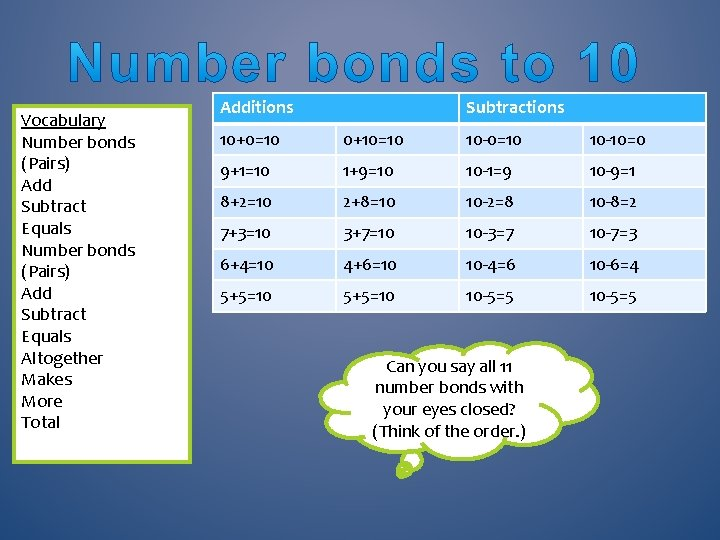Vocabulary Number bonds (Pairs) Add Subtract Equals Altogether Makes More Total Additions Subtractions 10+0=10 0+10=10 10 -10=0 9+1=10 1+9=10 10 -1=9 10 -9=1 8+2=10 2+8=10 10 -2=8 10 -8=2 7+3=10 3+7=10 10 -3=7 10 -7=3 6+4=10 4+6=10 10 -4=6 10 -6=4 5+5=10 10 -5=5 Can you say all 11 number bonds with your eyes closed? (Think of the order. )14 + 3 = 17 25 Tip: always start with the large number 63 Vocabulary Add on Equals Can you add 4 to 48?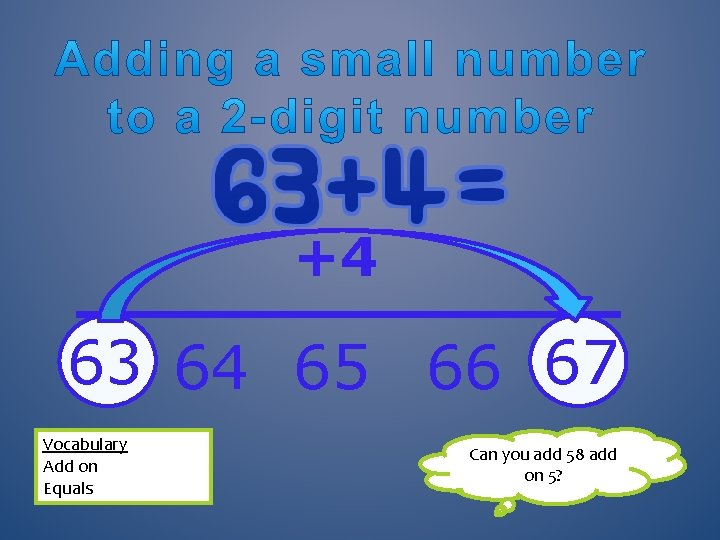+4 63 64 65 66 67 Vocabulary Add on Equals Can you add 58 add on 5?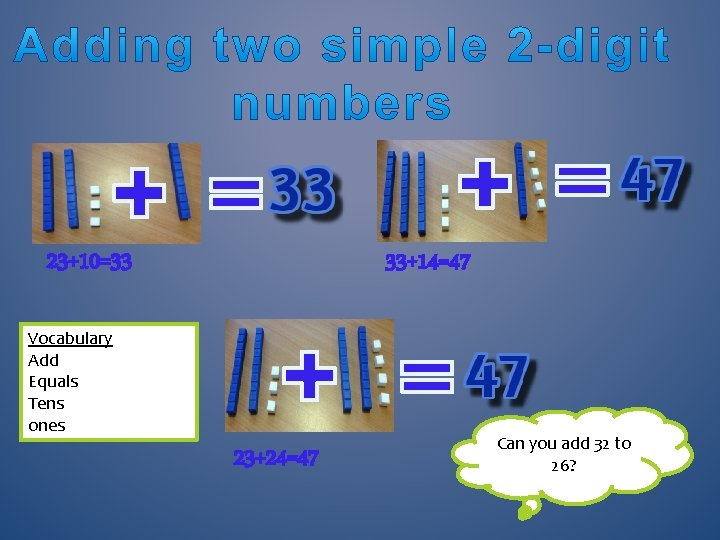23+10=33 33+14=47 Vocabulary Add Equals Tens ones 23+24=47 Can you add 32 to 26?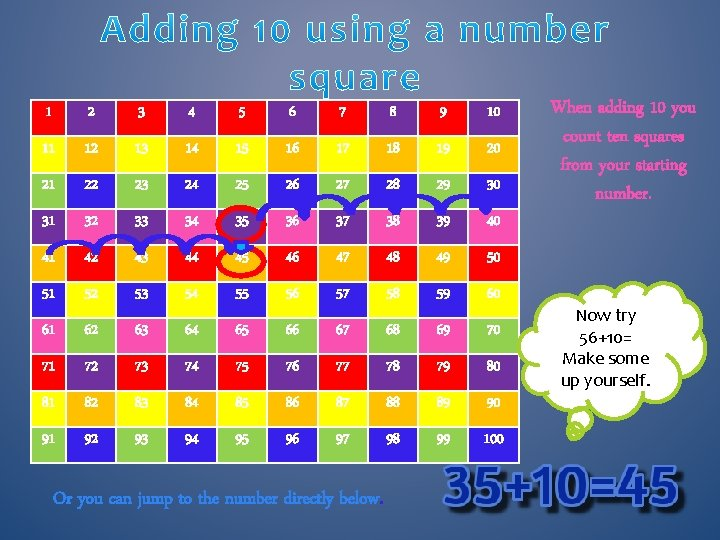1 2 3 4 5 6 7 8 9 10 11 12 13 14 15 16 17 18 19 20 21 22 23 24 25 26 27 28 29 30 31 32 33 34 35 36 37 38 39 40 41 42 43 44 45 46 47 48 49 50 51 52 53 54 55 56 57 58 59 60 61 62 63 64 65 66 67 68 69 70 71 72 73 74 75 76 77 78 79 80 81 82 83 84 85 86 87 88 89 90 91 92 93 94 95 96 97 98 99 100 Or you can jump to the number directly below. When adding 10 you count ten squares from your starting number. Now try 56+10= Make some up yourself.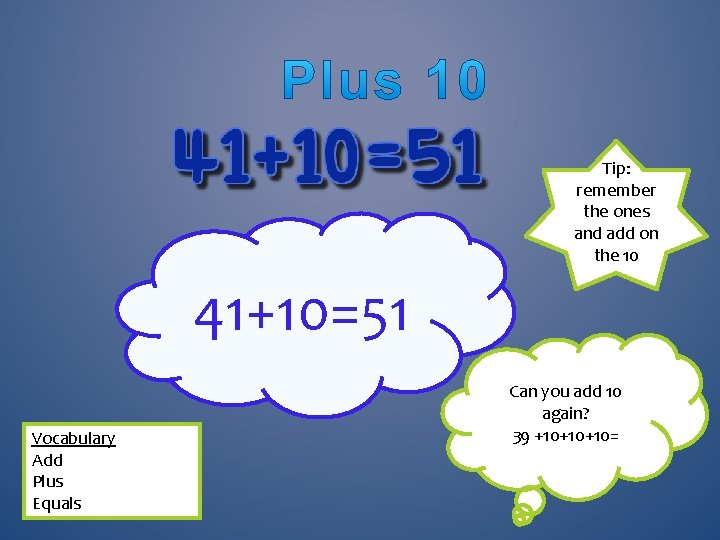Tip: remember the ones and add on the 10 41+10=51 Vocabulary Add Plus Equals Can you add 10 again? 39 +10+10+10=1 2 3 4 5 6 7 8 9 10 11 12 13 14 15 16 17 18 19 20 21 22 23 24 25 26 27 28 29 30 31 32 33 34 35 36 37 38 39 40 41 42 43 44 45 46 47 48 49 50 51 52 53 54 55 56 57 58 59 60 61 62 63 64 65 66 67 68 69 70 71 72 73 74 75 76 77 78 79 80 81 82 83 84 85 86 87 88 89 90 91 92 93 94 95 96 97 98 99 100 With this calculation you can add two 10 s (so 2 big jumps below). Then add 3 ones by jumping across 3. Tip: tens go up and down; ones go across Try: 46+32=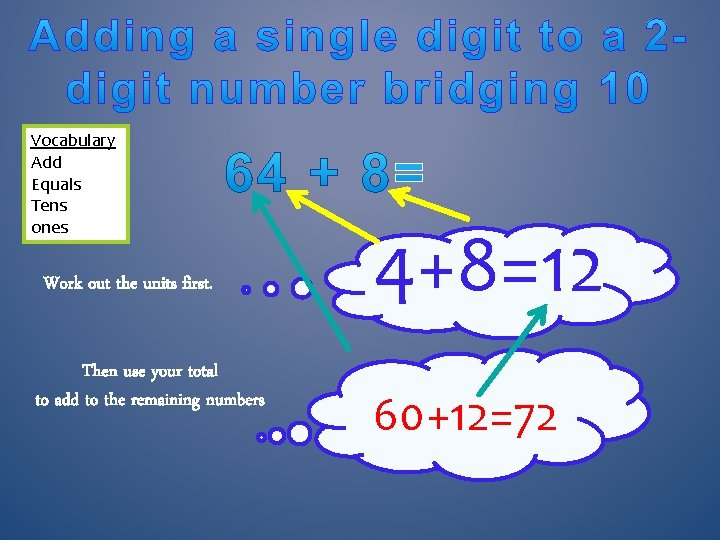Vocabulary Add Equals Tens ones Work out the units first. Then use your total to add to the remaining numbers 4+8=12 60+12=72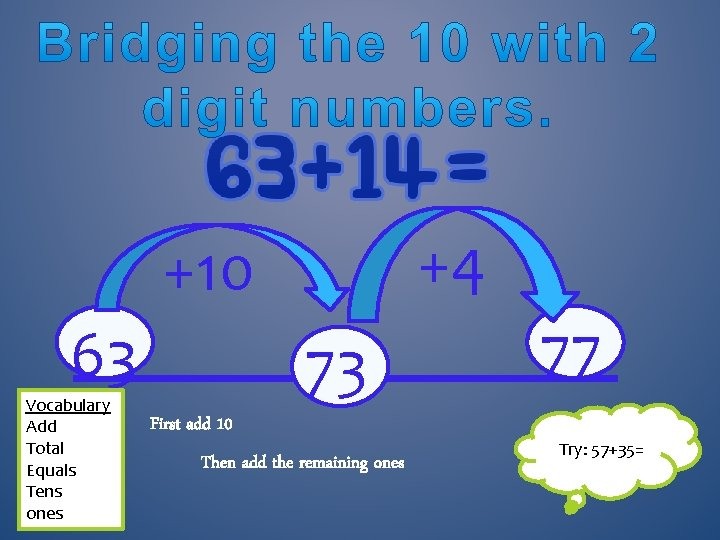+4 +10 63 Vocabulary Add Total Equals Tens ones 73 First add 10 Then add the remaining ones 77 Try: 57+35=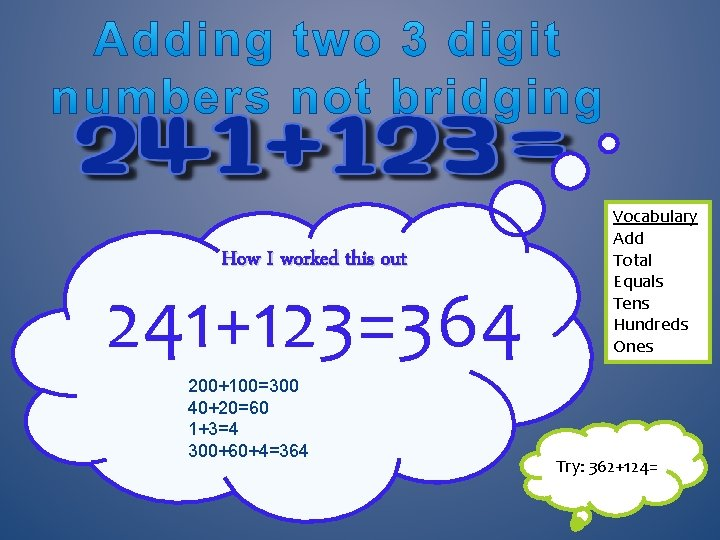How I worked this out 241+123=364 200+100=300 40+20=60 1+3=4 300+60+4=364 Vocabulary Add Total Equals Tens Hundreds Ones Try: 362+124=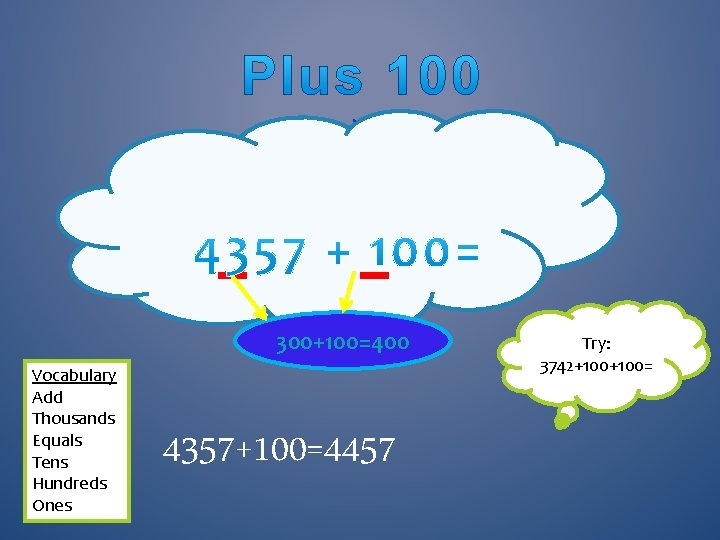. 300+100=400 Vocabulary Add Thousands Equals Tens Hundreds Ones 4357+100=4457 Try: 3742+100=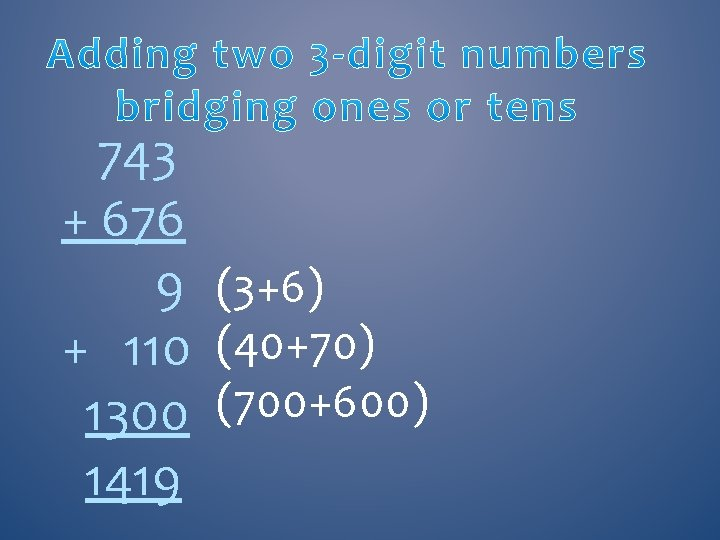743 + 676 9 (3+6) + 110 (40+70) 1300 (700+600) 1419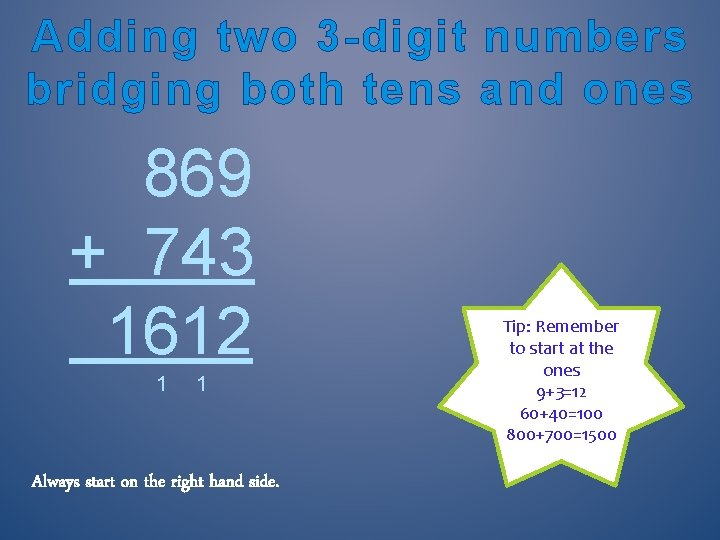Adding two 3 -digit numbers bridging both tens and ones 869 + 743 1612 1 1 Always start on the right hand side. Tip: Remember to start at the ones 9+3=12 60+40=100 800+700=1500Adding 2 numbers with 2 decimal points that bridge the tenths and hundredths 12. 53 + 9. 89 22. 42 1 1 How I worked this out: 1 Always start on the right hand side. 0. 03+0. 09=0. 12 0. 5+0. 8=1. 3 2+9+10=21 0. 12+1. 3+21=22. 42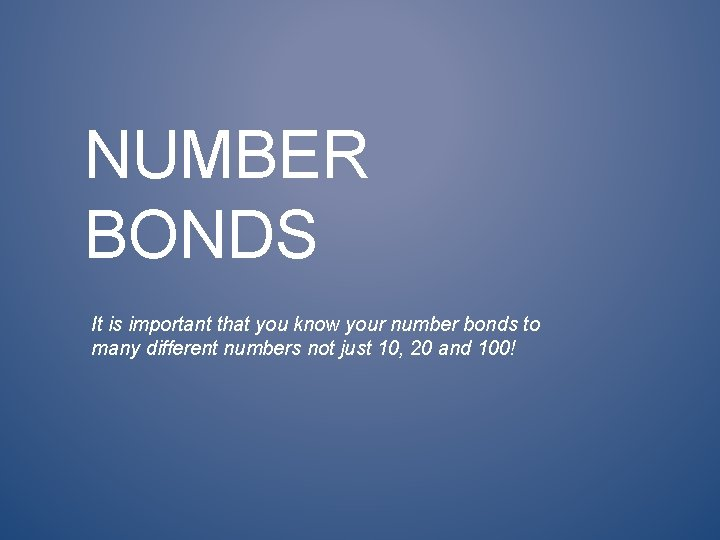NUMBER BONDS It is important that you know your number bonds to many different numbers not just 10, 20 and 100!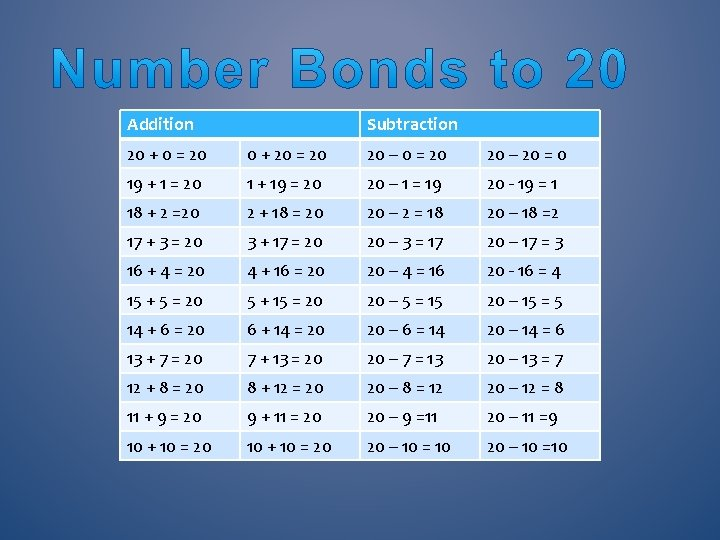Addition Subtraction 20 + 0 = 20 0 + 20 = 20 20 – 20 = 0 19 + 1 = 20 1 + 19 = 20 20 – 1 = 19 20 - 19 = 1 18 + 2 =20 2 + 18 = 20 20 – 2 = 18 20 – 18 =2 17 + 3 = 20 3 + 17 = 20 20 – 3 = 17 20 – 17 = 3 16 + 4 = 20 4 + 16 = 20 20 – 4 = 16 20 - 16 = 4 15 + 5 = 20 5 + 15 = 20 20 – 5 = 15 20 – 15 = 5 14 + 6 = 20 6 + 14 = 20 20 – 6 = 14 20 – 14 = 6 13 + 7 = 20 7 + 13 = 20 20 – 7 = 13 20 – 13 = 7 12 + 8 = 20 8 + 12 = 20 20 – 8 = 12 20 – 12 = 8 11 + 9 = 20 9 + 11 = 20 20 – 9 =11 20 – 11 =9 10 + 10 = 20 20 – 10 = 10 20 – 10 =10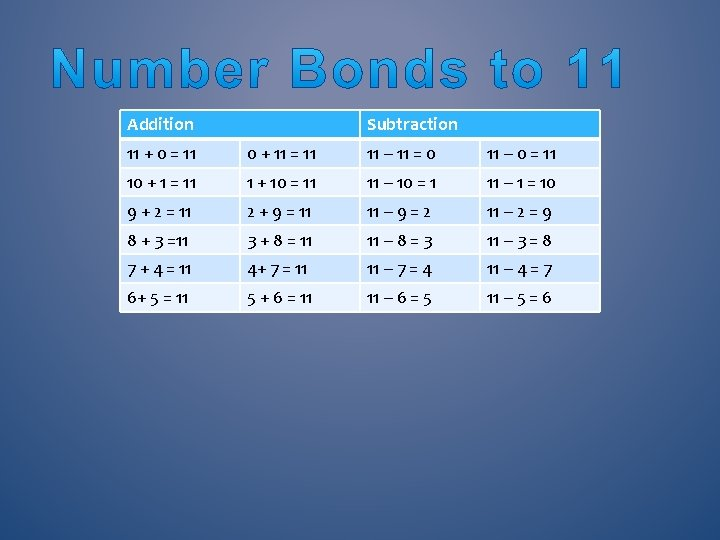Addition Subtraction 11 + 0 = 11 0 + 11 = 11 11 – 11 = 0 11 – 0 = 11 10 + 1 = 11 1 + 10 = 11 11 – 10 = 1 11 – 1 = 10 9 + 2 = 11 2 + 9 = 11 11 – 9 = 2 11 – 2 = 9 8 + 3 =11 3 + 8 = 11 11 – 8 = 3 11 – 3 = 8 7 + 4 = 11 4+ 7 = 11 11 – 7 = 4 11 – 4 = 7 6+ 5 = 11 5 + 6 = 11 11 – 6 = 5 11 – 5 = 6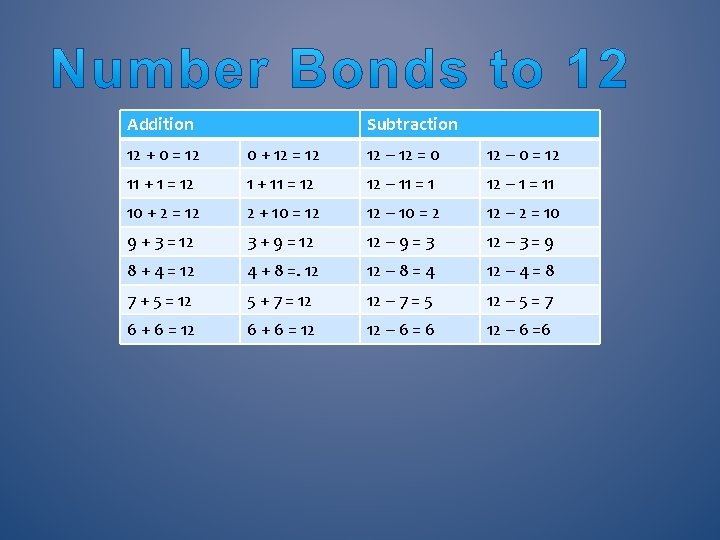Addition Subtraction 12 + 0 = 12 0 + 12 = 12 12 – 12 = 0 12 – 0 = 12 11 + 1 = 12 1 + 11 = 12 12 – 11 = 1 12 – 1 = 11 10 + 2 = 12 2 + 10 = 12 12 – 10 = 2 12 – 2 = 10 9 + 3 = 12 3 + 9 = 12 12 – 9 = 3 12 – 3 = 9 8 + 4 = 12 4 + 8 =. 12 12 – 8 = 4 12 – 4 = 8 7 + 5 = 12 5 + 7 = 12 12 – 7 = 5 12 – 5 = 7 6 + 6 = 12 12 – 6 = 6 12 – 6 =6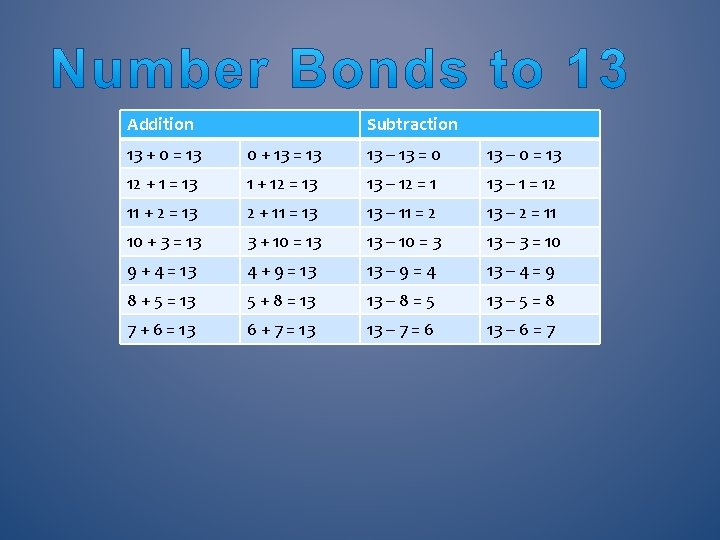Addition Subtraction 13 + 0 = 13 0 + 13 = 13 13 – 13 = 0 13 – 0 = 13 12 + 1 = 13 1 + 12 = 13 13 – 12 = 1 13 – 1 = 12 11 + 2 = 13 2 + 11 = 13 13 – 11 = 2 13 – 2 = 11 10 + 3 = 13 3 + 10 = 13 13 – 10 = 3 13 – 3 = 10 9 + 4 = 13 4 + 9 = 13 13 – 9 = 4 13 – 4 = 9 8 + 5 = 13 5 + 8 = 13 13 – 8 = 5 13 – 5 = 8 7 + 6 = 13 6 + 7 = 13 13 – 7 = 6 13 – 6 = 7Addition Subtraction 14 + 0 =14 0 + 14 = 14 14 – 0 = 14 14 – 14 = 0 13 + 1 = 14 1 + 13 = 14 14 – 1 = 13 14 – 13 = 1 12 + 2 = 14 2 + 12 = 14 14 – 2 = 12 14 – 12 = 2 11 + 3 = 14 3 + 11 = 14 14 – 3 = 11 14 – 11 = 3 10 + 4 = 14 4 + 10 = 14 14 – 4 = 10 14 – 10 = 4 9 + 5 = 14 5 + 9 = 14 14 – 5 = 9 14 – 9 = 5 8 + 6 = 14 6 + 8 = 14 14 – 6 = 8 14 – 8 = 6 7 + 7 = 14 14 – 7 =7 14 – 7 = 7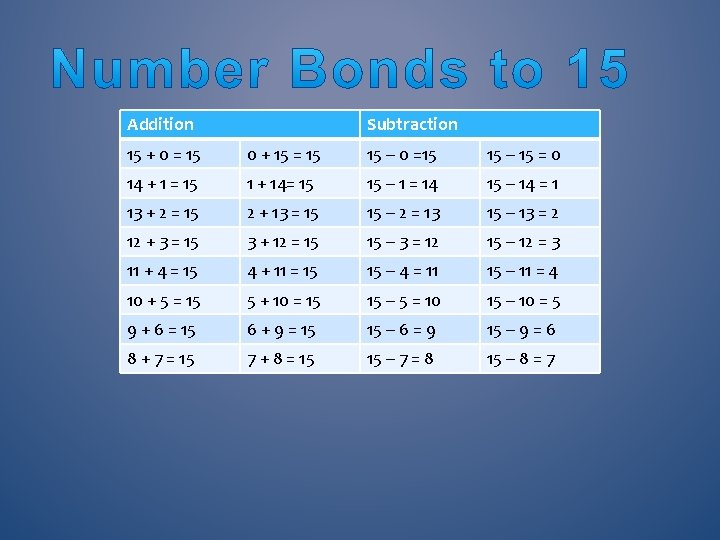Addition Subtraction 15 + 0 = 15 0 + 15 = 15 15 – 0 =15 15 – 15 = 0 14 + 1 = 15 1 + 14= 15 15 – 1 = 14 15 – 14 = 1 13 + 2 = 15 2 + 13 = 15 15 – 2 = 13 15 – 13 = 2 12 + 3 = 15 3 + 12 = 15 15 – 3 = 12 15 – 12 = 3 11 + 4 = 15 4 + 11 = 15 15 – 4 = 11 15 – 11 = 4 10 + 5 = 15 5 + 10 = 15 15 – 5 = 10 15 – 10 = 5 9 + 6 = 15 6 + 9 = 15 15 – 6 = 9 15 – 9 = 6 8 + 7 = 15 7 + 8 = 15 15 – 7 = 8 15 – 8 = 7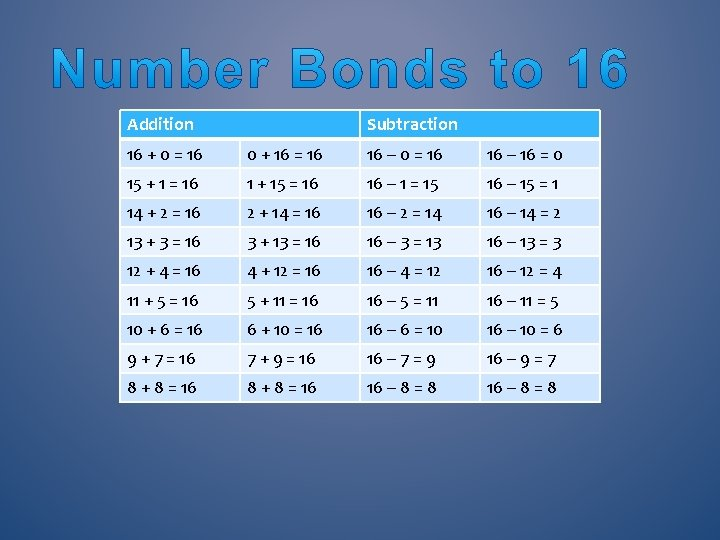Addition Subtraction 16 + 0 = 16 0 + 16 = 16 16 – 0 = 16 16 – 16 = 0 15 + 1 = 16 1 + 15 = 16 16 – 1 = 15 16 – 15 = 1 14 + 2 = 16 2 + 14 = 16 16 – 2 = 14 16 – 14 = 2 13 + 3 = 16 3 + 13 = 16 16 – 3 = 13 16 – 13 = 3 12 + 4 = 16 4 + 12 = 16 16 – 4 = 12 16 – 12 = 4 11 + 5 = 16 5 + 11 = 16 16 – 5 = 11 16 – 11 = 5 10 + 6 = 16 6 + 10 = 16 16 – 6 = 10 16 – 10 = 6 9 + 7 = 16 7 + 9 = 16 16 – 7 = 9 16 – 9 = 7 8 + 8 = 16 16 – 8 = 8Addition Subtraction 17 + 0 = 17 0 + 17 = 17 17 – 0 = 17 17 – 17 = 0 16 + 1 = 17 1 + 16 = 17 17 – 1 = 16 17 – 16 = 1 15 + 2 = 17 2 + 15 = 17 17 – 2 = 15 17 – 15 = 2 14 + 3 = 17 3 + 14 = 17 17 – 3 = 14 17 – 14 = 3 13 + 4 = 17 4 + 13 = 17 17 – 4 = 13 17 – 13 = 4 12 + 5 = 17 5 + 12 = 17 17 – 5 = 12 17 – 12 = 5 11 + 6 = 17 6 + 11 = 17 17 – 6 = 11 17 – 11 = 6 10 + 7 = 17 7 + 10 = 17 17 – 7 = 10 17 – 10 = 7 9 + 8 = 17 8 + 9 = 17 17 – 8 = 9 17 – 9 = 8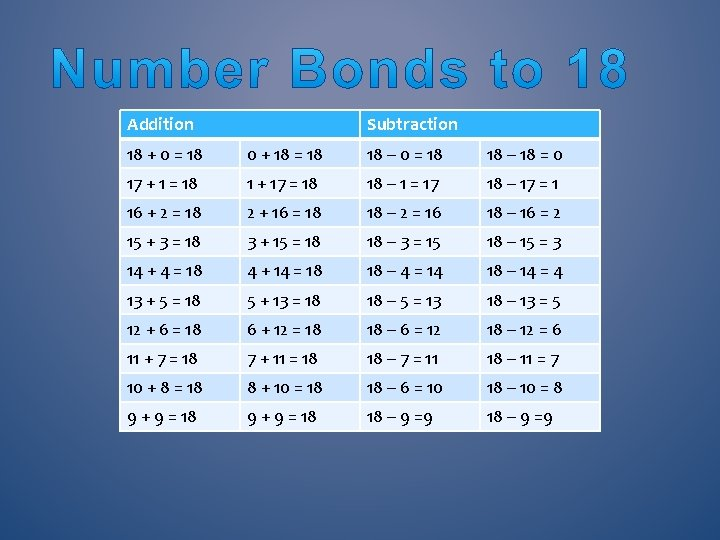Addition Subtraction 18 + 0 = 18 0 + 18 = 18 18 – 0 = 18 18 – 18 = 0 17 + 1 = 18 1 + 17 = 18 18 – 1 = 17 18 – 17 = 1 16 + 2 = 18 2 + 16 = 18 18 – 2 = 16 18 – 16 = 2 15 + 3 = 18 3 + 15 = 18 18 – 3 = 15 18 – 15 = 3 14 + 4 = 18 4 + 14 = 18 18 – 4 = 14 18 – 14 = 4 13 + 5 = 18 5 + 13 = 18 18 – 5 = 13 18 – 13 = 5 12 + 6 = 18 6 + 12 = 18 18 – 6 = 12 18 – 12 = 6 11 + 7 = 18 7 + 11 = 18 18 – 7 = 11 18 – 11 = 7 10 + 8 = 18 8 + 10 = 18 18 – 6 = 10 18 – 10 = 8 9 + 9 = 18 18 – 9 =9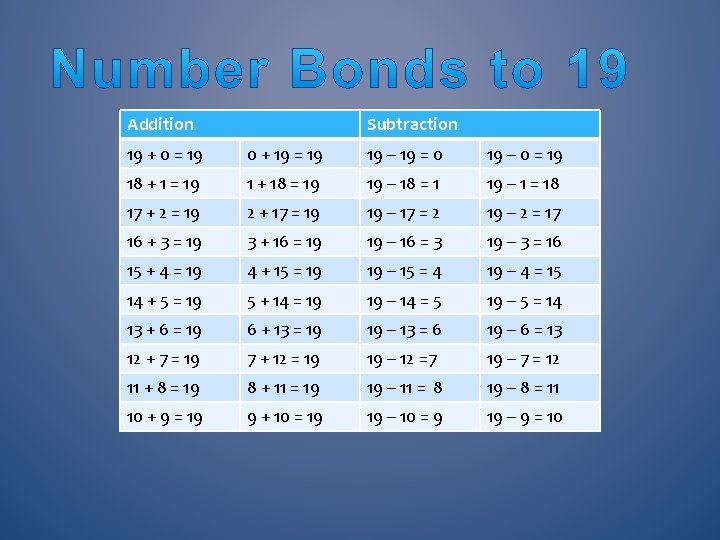Addition Subtraction 19 + 0 = 19 0 + 19 = 19 19 – 19 = 0 19 – 0 = 19 18 + 1 = 19 1 + 18 = 19 19 – 18 = 1 19 – 1 = 18 17 + 2 = 19 2 + 17 = 19 19 – 17 = 2 19 – 2 = 17 16 + 3 = 19 3 + 16 = 19 19 – 16 = 3 19 – 3 = 16 15 + 4 = 19 4 + 15 = 19 19 – 15 = 4 19 – 4 = 15 14 + 5 = 19 5 + 14 = 19 19 – 14 = 5 19 – 5 = 14 13 + 6 = 19 6 + 13 = 19 19 – 13 = 6 19 – 6 = 13 12 + 7 = 19 7 + 12 = 19 19 – 12 =7 19 – 7 = 12 11 + 8 = 19 8 + 11 = 19 19 – 11 = 8 19 – 8 = 11 10 + 9 = 19 9 + 10 = 19 19 – 10 = 9 19 – 9 = 10Addition Subtraction 100 + 0 = 100 0 + 100 = 100 – 100 = 0 100 – 0 = 100 90 + 10 = 100 10 + 90 = 100 – 90 = 10 100 – 10 = 90 80 + 20 = 100 20 + 80 = 100 – 80 = 20 100 – 20 = 80 70 + 30 = 100 30 + 70 = 100 – 70 = 30 100 – 30 = 70 60 + 40 = 100 40 + 60 = 100 – 60 = 40 100 – 40 = 60 50 + 50 = 100 100 – 50 = 50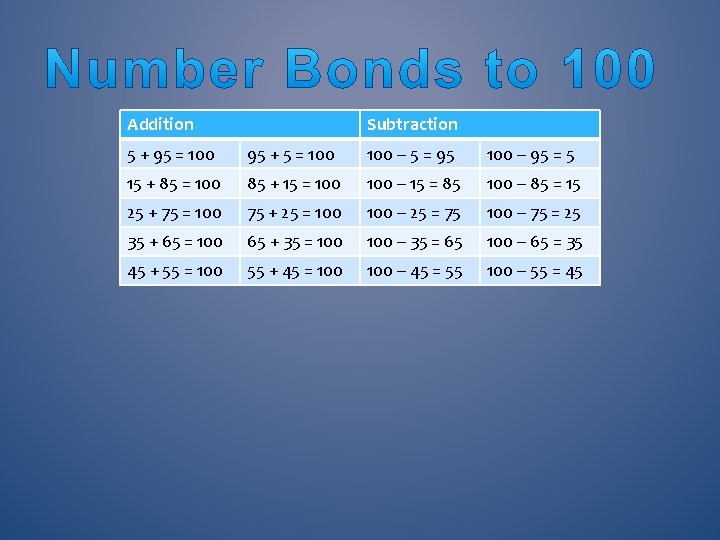Addition Subtraction 5 + 95 = 100 95 + 5 = 100 – 5 = 95 100 – 95 = 5 15 + 85 = 100 85 + 15 = 100 – 15 = 85 100 – 85 = 15 25 + 75 = 100 75 + 25 = 100 – 25 = 75 100 – 75 = 25 35 + 65 = 100 65 + 35 = 100 – 35 = 65 100 – 65 = 35 45 + 55 = 100 55 + 45 = 100 – 45 = 55 100 – 55 = 45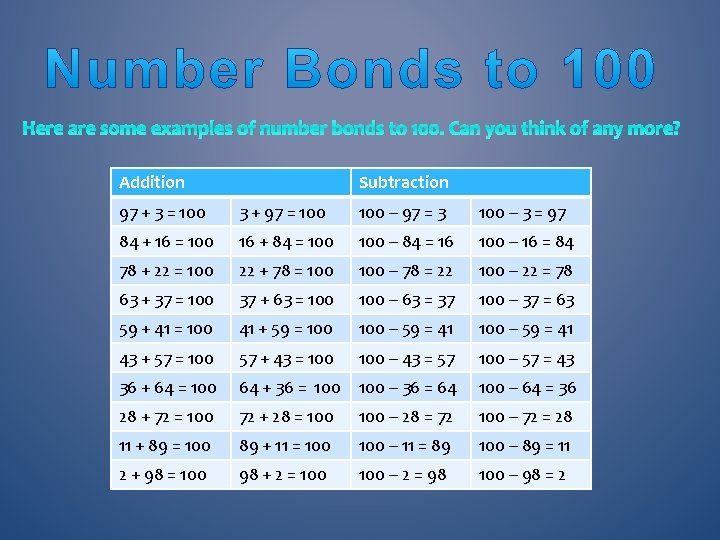Here are some examples of number bonds to 100. Can you think of any more? Addition Subtraction 97 + 3 = 100 3 + 97 = 100 – 97 = 3 100 – 3 = 97 84 + 16 = 100 16 + 84 = 100 – 84 = 16 100 – 16 = 84 78 + 22 = 100 22 + 78 = 100 – 78 = 22 100 – 22 = 78 63 + 37 = 100 37 + 63 = 100 – 63 = 37 100 – 37 = 63 59 + 41 = 100 41 + 59 = 100 – 59 = 41 43 + 57 = 100 57 + 43 = 100 – 43 = 57 100 – 57 = 43 36 + 64 = 100 64 + 36 = 100 – 36 = 64 100 – 64 = 36 28 + 72 = 100 72 + 28 = 100 – 28 = 72 100 – 72 = 28 11 + 89 = 100 89 + 11 = 100 – 11 = 89 100 – 89 = 11 2 + 98 = 100 98 + 2 = 100 – 2 = 98 100 – 98 = 223+10=33 23+24=4770 p+30 p=£ 1. 00 40 p+60 p=£ 1. 00 90 p+10 p=£ 1. 00 80 p+20 p=£ 1. 00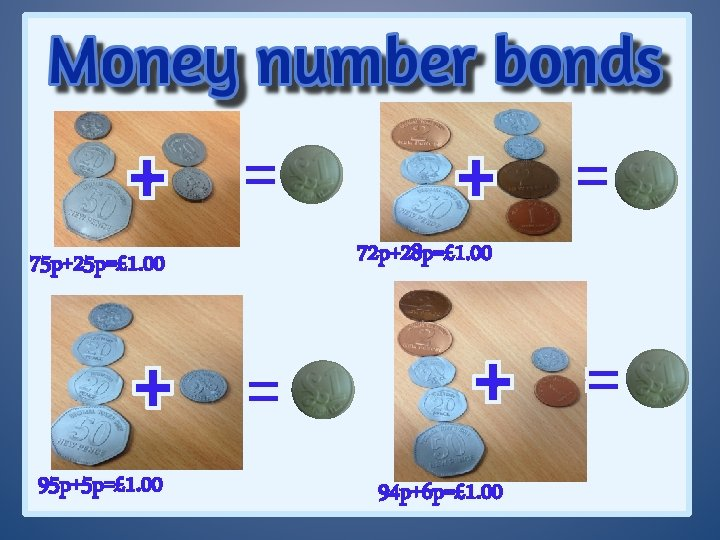75 p+25 p=£ 1. 00 95 p+5 p=£ 1. 00 72 p+28 p=£ 1. 00 94 p+6 p=£ 1. 00